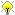[Documentation] [TitleIndex] [WordIndex]Please ask about problems and questions regarding this tutorial on answers.ros.org. Don't forget to include in your question the link to this page, the versions of your OS & ROS, and also add appropriate tags.

# Frame transformations (Python)

Description: This tutorials gets you started with KDL Frames, Vectors, Rotations, etc

Tutorial Level:

## Data types

Creating a Frame, Vector and Rotation

```   1 import PyKDL
2
3 # create a vector
4 v = PyKDL.Vector(1,3,5)
5
6 # create a rotation from Roll Pitch, Yaw angles
7 r1 = PyKDL.Rotation.RPY(1.2, 3.4, 0)
8
9 # create a rotation from XYZ Euler angles
10 r2 = PyKDL.Rotation.EulerZYX(0, 1, 0)
11
12 # create a rotation from a rotation matrix
13 r3 = PyKDL.Rotation(1,0,0, 0,1,0, 0,0,1)
14
15 # create a frame from a vector and a rotation
16 f = PyKDL.Frame(r2, v)
```

Extracting information from a Frame, Vector and Rotation

```   1 import PyKDL
2
3 # frame
4 f = PyKDL.Frame(PyKDL.Rotation.RPY(0,1,0),
5                 PyKDL.Vector(3,2,4))
6
7 # get the origin (a Vector) of the frame
8 origin = f.p
9
10 # get the x component of the origin
11 x = origin.x()
12 x = origin
13
14 # get the rotation of the frame
15 rot = f.M
16
17 # get ZYX Euler angles from the rotation
18 [Rz, Ry, Rx] = rot.GetEulerZYX()
19
20 # get the RPY (fixed axis) from the rotation
21 [R, P, Y] = rot.GetRPY()
```

## Creating from ROS types

```   1 from tf_conversions import posemath
2
3 # you have a Pose message
4 pose = Pose()
5
6 # convert the pose into a kdl frame
7 f1 = posemath.fromMsg(pose)
8
9 # create another kdl frame
10 f2 = PyKDL.Frame(PyKDL.Rotation.RPY(0,1,0),
11                  PyKDL.Vector(3,2,4))
12
13 # Combine the two frames
14 f = f1 * f2
15
16 # and convert the result back to a pose message
17 pose = toMsg(f)
```

And it wouldn't be Python if you couldn't have done all this in a single line:

```   1 from tf_conversions import posemath
2
3 pose = posemath.toMsg(posemath.fromMsg(pose) * PyKDL.Frame(PyKDL.Rotation.RPY(0,1,0), PyKDL.Vector(3,2,4)))
```

## Transformations

Transforming a point

```   1 import PyKDL
2
3 # define a frame
4 f = PyKDL.Frame(PyKDL.Rotation.RPY(0,1,0),
5                 PyKDL.Vector(3,2,4))
6
7 # define a point
8 p = PyKDL.Vector(1, 0, 0)
9 print p
10
11 # transform this point with f
12 p = f * p
13 print p
```

2019-11-09 12:52